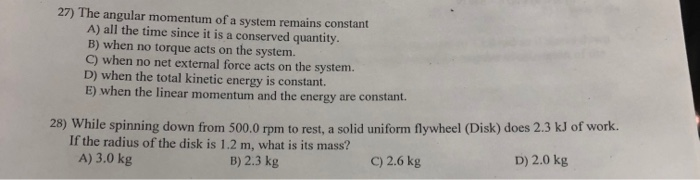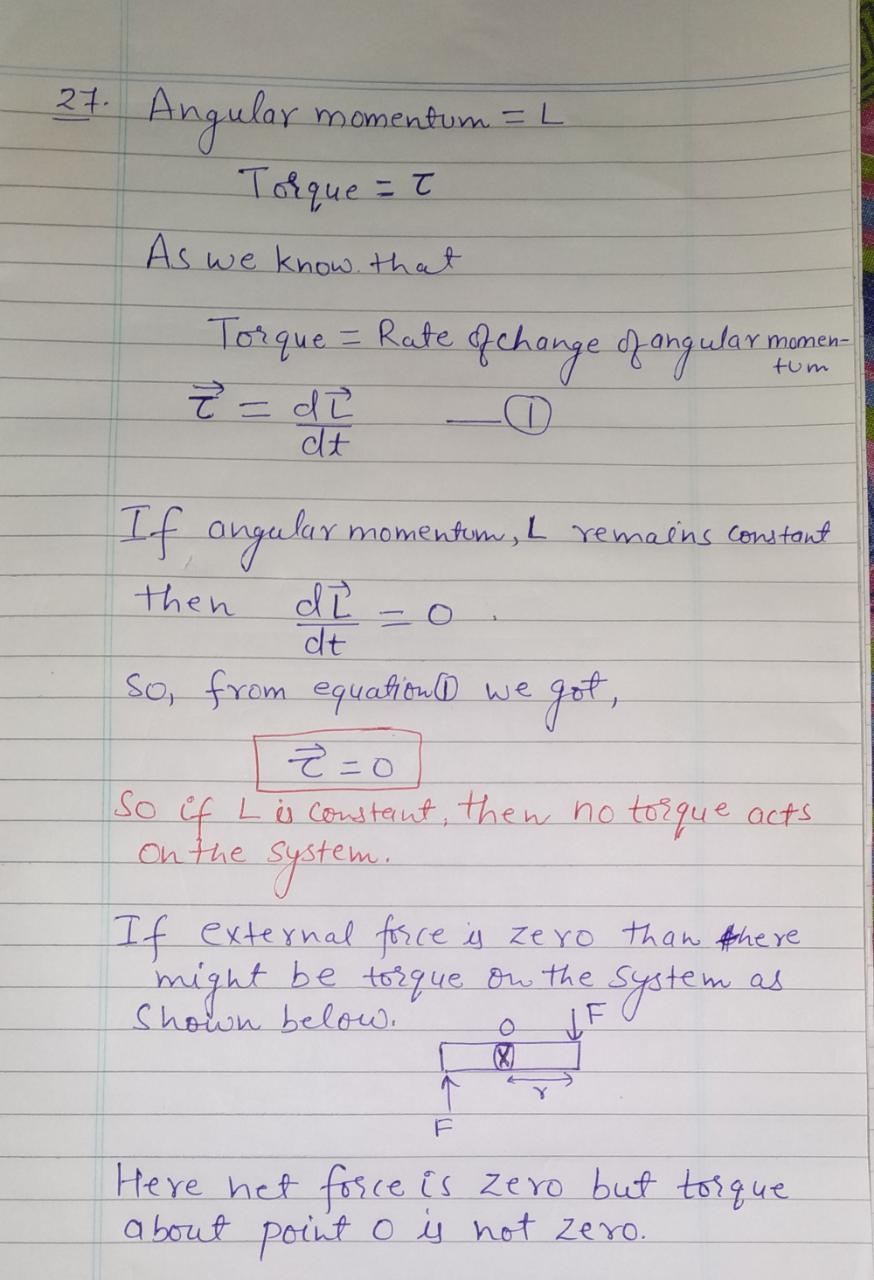# The angular momentum of a system remains constant27) The angular momentum of a system remains constant A) all the time since it is a conserved quantity. B) when no torque acts on the system. C) when no net external force acts on the system. D) when the total kinetic energy is constant. E) when the linear momentum and the energy are constant. 28) While spinning down from 500.0 rpm to rest, a solid uniform flywheel (Disk) does 2.3 kJ of work. If the radius of the disk is 1.2 m, what is its mass? A) 3.0 kg D) 2.0 kg C) 2.6 kg B) 2.3 kg

Option B is correct when no torque acts on
the system.
Let me know if you need any further clarification on this.
Please upvote the answer if you have understood the solution
properly.
21 Angular Totgue = T momentum L As we know that Torque = Rate Rehange qanguts Y momen- tum momentum, L remains Censtant then dt So, frem equatitD we So if Lu Cewteut then no toque acts On the If external frce a zero than fhe ye be torgue Bre the yetem as Shown belo Here het a bout poit fosce is zero but toigue o not Zero.